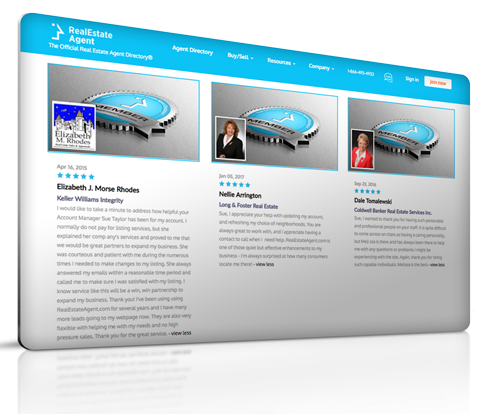# Accumulated Depreciation

## Definition of "Accumulated Depreciation"

Sharon Dolezal, Real Estate Agent Coldwell Banker

Any real estate owner is aware that assets depreciate over time. So exactly what is accumulated depreciation then? As depreciation happens to every asset, whether it is a three-story mansion or a couch. The accumulated depreciation definition explains how this type of depreciation calculates the asset’s depreciation up to a specific point in its life. Accumulated appreciation works to determine the contra asset account, or in other words, the natural balance that lowers the asset value. It is also referred to as the accrual of depreciation as it uses the accrual method of accounting for expenses and revenues.

### How does Accumulated Depreciation work?

Any business that adheres to the generally accepted accounting principles (GAAP) respects the matching principle that demands matching each revenue in the same accounting period with its corresponding expense. The business expense is the capital asset’s value during every year through depreciation, but in that same period, those assets generate revenue while they are used. The expense and the revenue will match up and be added through adjusting journal entries.

Accumulated depreciation summarizes how much an asset depreciated up to a certain point. After each accounting period, the depreciation expense is added to the already existing accumulated depreciation in the books. The asset’s value that depreciated at the end of its useful life is its carrying value. When the asset is no longer useful, that value will match its salvage value, which is the revenue gained from the use of that asset. The carrying value is the asset’s original cost minus its accumulated depreciation.

### Example of Accumulated Depreciation

Purchasing a piece of equipment for \$11,000 with a useful life expectancy of 10 years and a salvage value of \$1,000, the company splits the equipment’s cost for the following 10 years. The company will depreciate at an amount of \$1,000 yearly until the value in the books for the asset is at \$1,000. After each accounting period is over, the new value of depreciation is added to the one before. Each year the depreciation accumulates, and the accumulated depreciation grows by \$1,000 every year for the following 10 years. At the end of the asset’s useful life expectancy, the accumulated depreciation will be \$10,000, and its carrying value matches with its salvage value.

### Are you a realtor and want to become a member?

Residential Rental Commercial

#### Have a question or comment? We're here to help.